# Is there a way to determine the maximum inscribed circle within a particle count in Image-Pro 11?

Is there a Measurement List Types option in Image-Pro 11 that allows for the determination of the maximum inscribed circle within particle count?

• Hi uaya,

If you want to create the circle manually, you can use Best-Fit circle tool.
If you want to get it automatically, you can use statistical table to get min/max values of object centroids and then draw the circle based on the bounding box of all objects from a macro.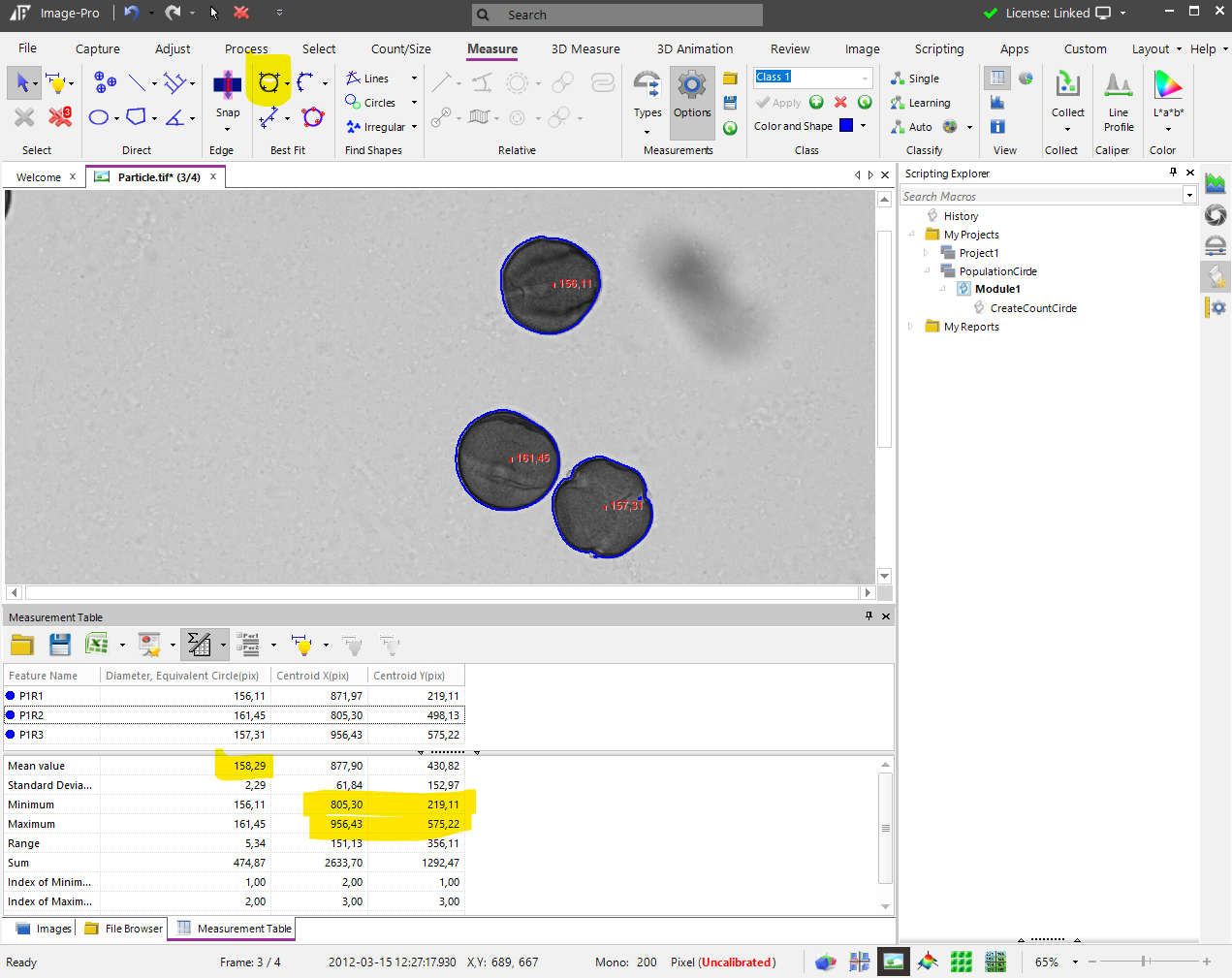Here is the macro that can draw the circle inscribing all objects inside:
```Imports MediaCy.Addins.Measurements
Public Module Module1

Public Sub CreateCountCircle
Dim im As McImage=ThisApplication.ActiveImage
If im Is Nothing Then Exit Sub
Dim md As McMMData=im.MeasurementsData
If md.Count=0 Then Exit Sub
Dim pixSize As Double=1
If im.SpatialCalibration IsNot Nothing Then
'get pixel size of calibrated image
pixSize=im.SpatialCalibration.PixelSizeX
End If
'get max/min of CentroidX and CentroidY
Dim xmin As Double=md.Statistics(eMeasures.RgnCentroidX).Minimum
Dim xmax As Double=md.Statistics(eMeasures.RgnCentroidX).Maximum
Dim ymin As Double=md.Statistics(eMeasures.RgnCentroidY).Minimum
Dim ymax As Double=md.Statistics(eMeasures.RgnCentroidY).Maximum
'get average object diameter
Dim dmean As Double=md.Statistics(eMeasures.RgnDiameterEq).Mean
'calculate circle center in pixel coordinates
Dim x As Double=((xmin+xmax)/2)/pixSize
Dim y As Double=((ymin+ymax)/2)/pixSize
'calculate diameter x and y
Dim dx As Double=xmax-xmin
Dim dy As Double=ymax-ymin
'get Max radius on inscribed circle (including dmean)
Dim r As Double=((System.Math.Max(dx,dy)+dmean)/2)/pixSize
'draw circle
Dim meas1
.MeasurementType = McMeasurements.enumMMSTypes.mmtsEllipse
.Points = New System.Collections.Generic.List(Of System.Drawing.PointF)
.FeatureName = "Count Circle"
.SnapFeature = False
.Run(im, meas1)
End With
End Sub
End Module
```
The result will look like this: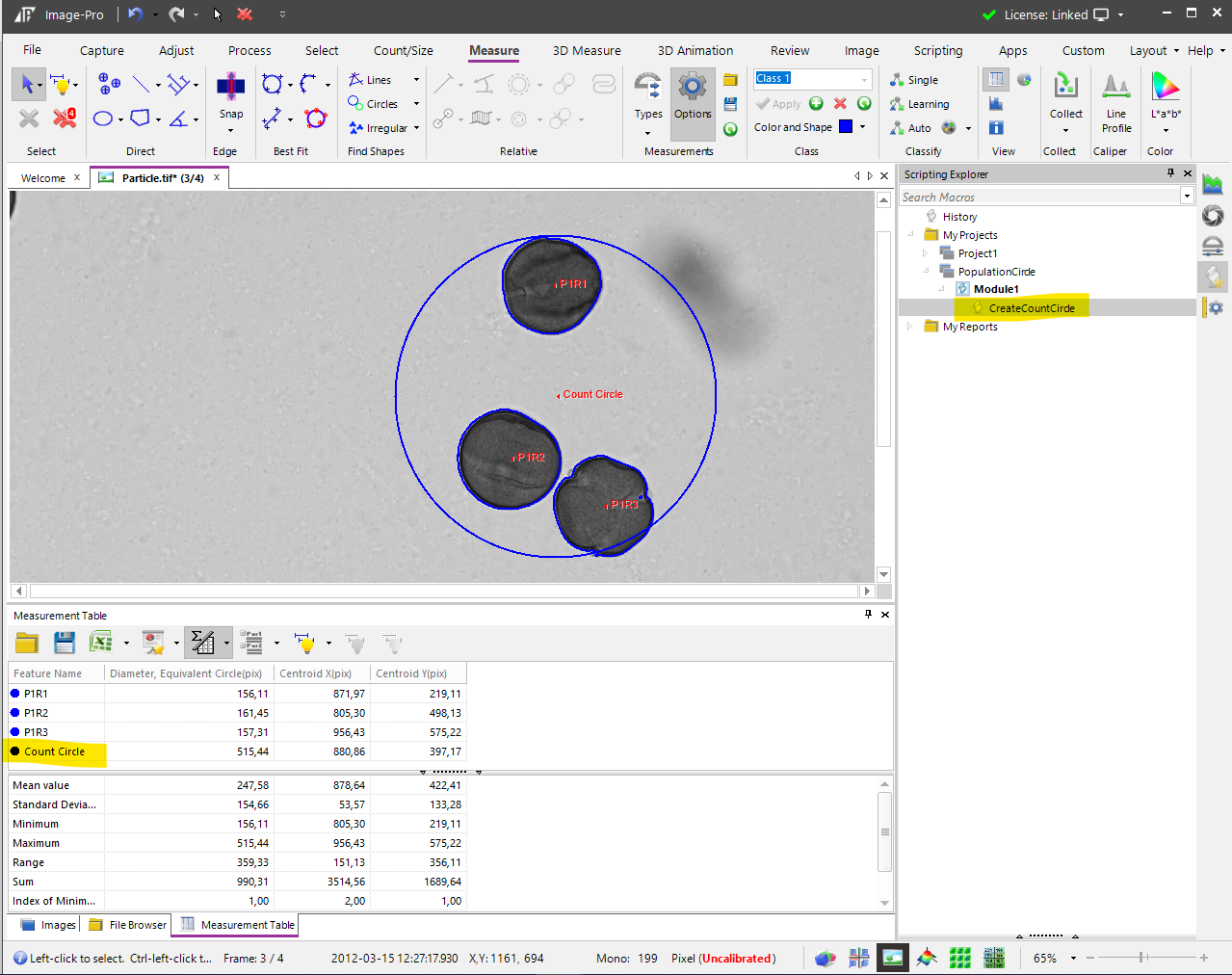Regards,

Yuri
• Thank you for your prompt reply. To clarify, I'm interested in finding the maximum inscribed circle within each individual particle, as depicted in the image. So, for a scenario with three particles, I aim to identify one inscribed circle within each particle, resulting in a total of three inscribed circles.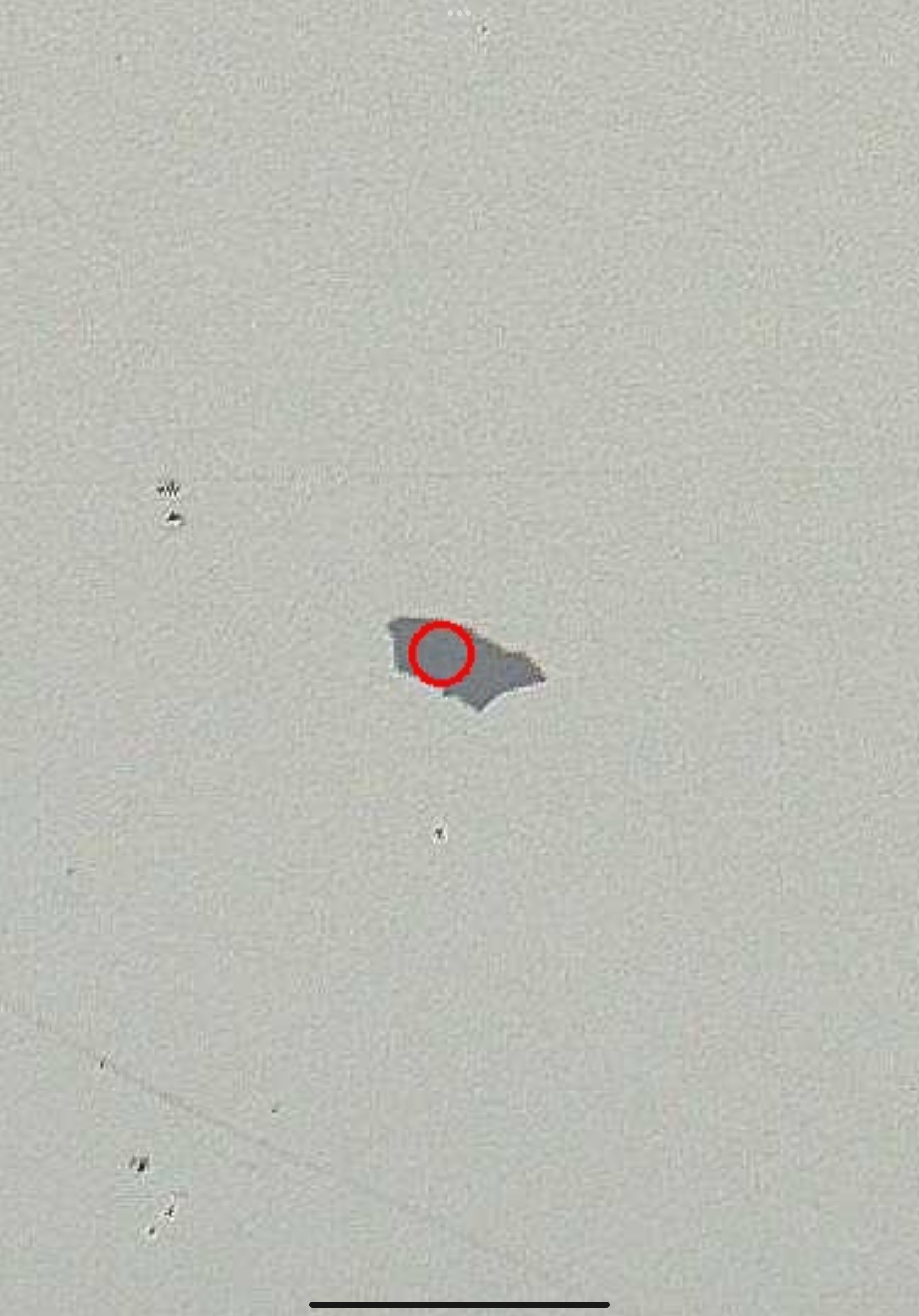• Hi uaya,

Then I completely misunderstood your question. Measuring inscribed circle of every measured object is a different task.

There is no built-in measurement that provides this measure, but I will show you the steps how to get it using a Distance map. Distance map creates an image of the mask where pixel intensities are equal to the distance from the current pixel to the closest edge of the object, so measuring the Maximum Intensity of the distance map will give you the Radius of the Inscribed circle to that object, in pixels.

Here are the steps: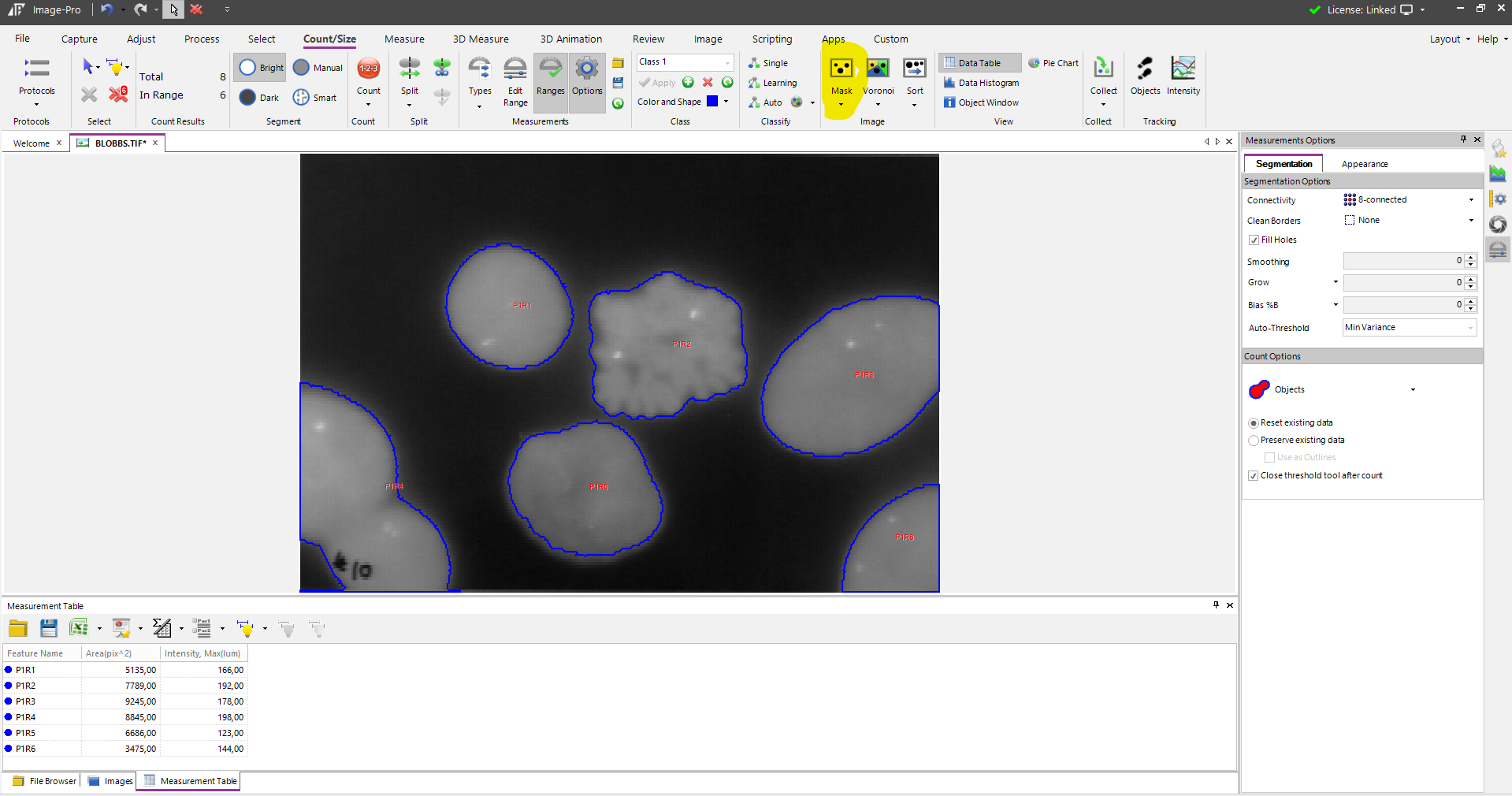2. Create Distance Map of the mask image, use 3D Filters, as they produce a higher accuracy map: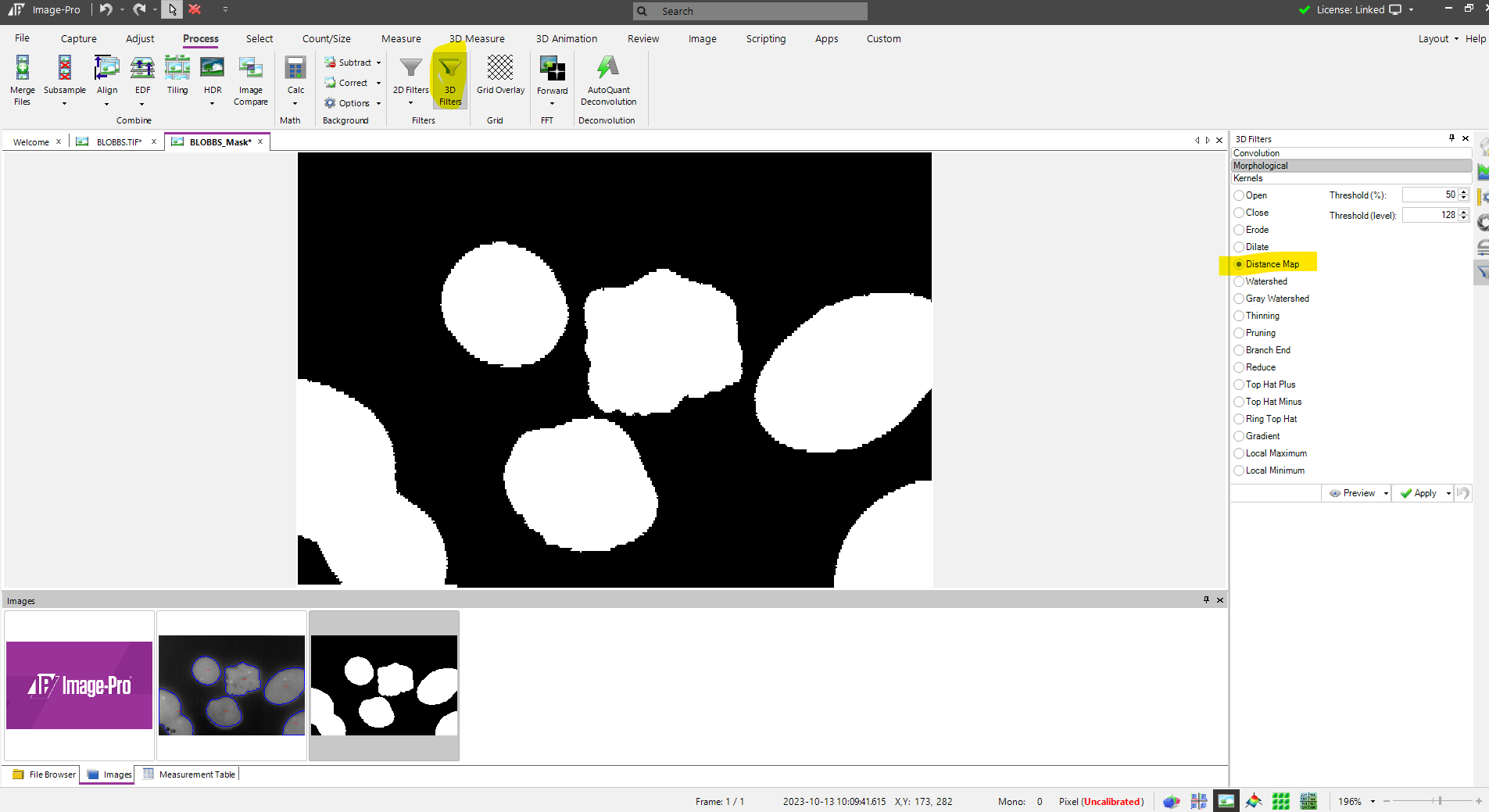3. Segment distance map using Threshold=1 and measure Intensity Max of each object - it corresponds to the Radius of the inscribed circle (in pixels)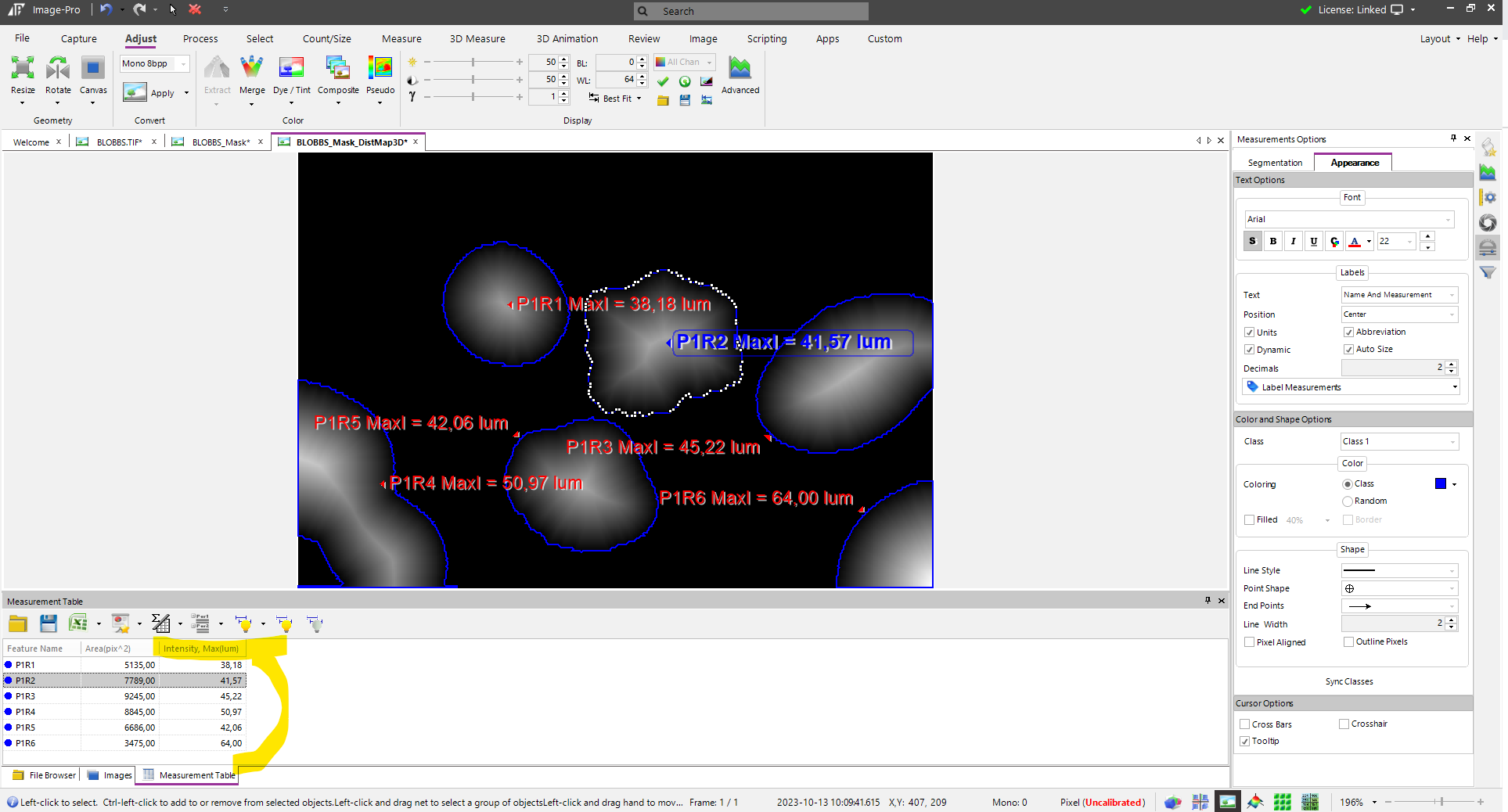4. Optionally, if your image is calibrated, you can create Intensity calibration that matches the spatial calibration, so Intensity Max will give you the Radius in Calibrated units (µm).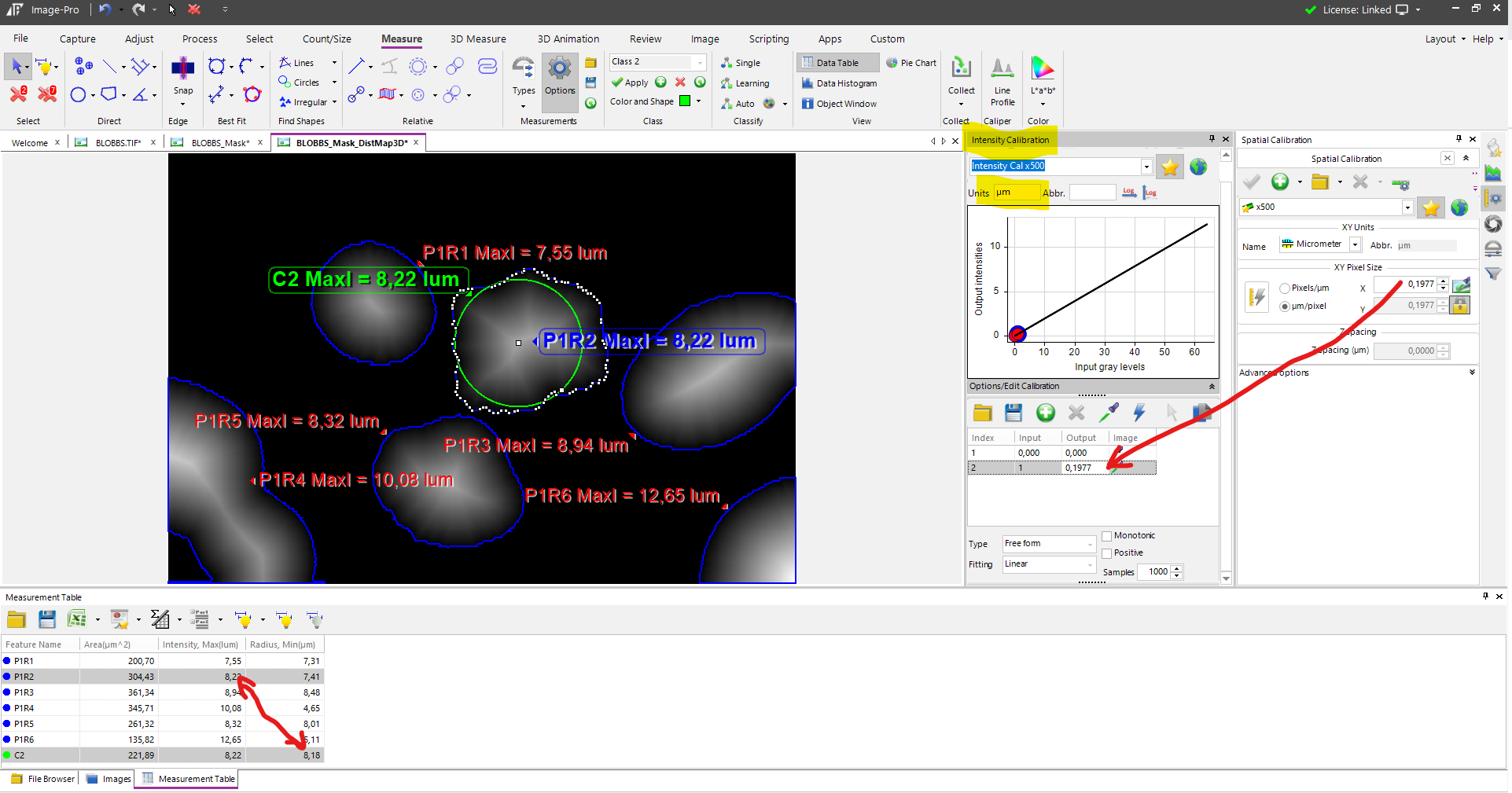I've added an Inscribed Circle (C2) to the image, that illustrates that the Radius of the circle matches the Intensity Max of the object, in micrometers.

Yuri
• If you are OK with the smallest inscribed circle centered at the object Centroid, you could use the Diameter, Min measurement.  Then your result would be a circle centered at the CentroidX, CentroidY with radius equal to one half of the Diameter, Min.  Yuri's approach will work for any object shape, but you will need to find the location of the distance map maxima to find the centers of your inscribed circles (the value of the maxima is the radius, in pixels).
• Whoops, my last post was misleading.  The center of the inscribed circle would be the average of the two end-points of the minimum diameter line, which passes through the centroid but is not necessarily evenly balanced around it.  To find the end points of the minimum diameter line, you would need to find the minimum diameter in the Diameters array and then get the two radii corresponding to that diameter from the Radii array.  At this point it might be less work to use Yuri's distance map approach.  However, if all you need is an estimate of the size of the smallest inscribed circle, the Diameter, Min measurement would give you that directly without any extra computation.
• YuriG, I had already calculated the maximum inscribed circle's diameter in Python before I saw your solution. However, I've now implemented your solution on one image, and the results appear quite similar. I plan to assess its performance across multiple images and make a comparison. I'm leaning towards using ImagePro for its Measurement List Types feature to gather additional data. Your solution was greatly appreciated.

CraigW, I initially experimented with the Minimum Diameter and Minor Axis methods prior to posting this question on the forum. The Minimum Diameter values turned out to be significantly smaller, while the Minor Axis values were slightly larger when compared to the diameter of the maximum inscribed circle. Thanks for your input.